Example problem: Adaptive simulation of finite-Reynolds number entry flow into a 3D tube

In this example we shall demonstrate the spatially adaptive solution of the steady 3D Navier-Stokes equations using the problem of developing pipe flow.

# The example problem

 The 3D developing pipe flow in a quarter-tube domain. Solve the steady Navier-Stokes equations: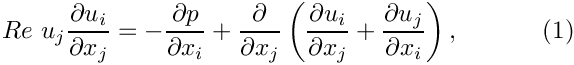and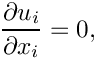in the quarter-tube domain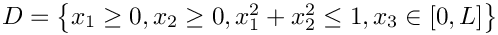, subject to the Dirichlet boundary conditions: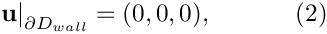on the curved wall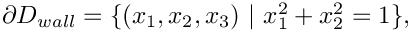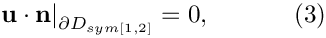(where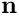is the outer unit normal vector) on the symmetry boundaries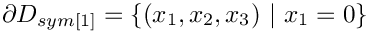and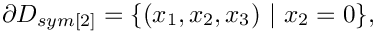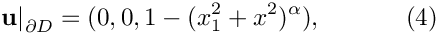on the inflow boundary,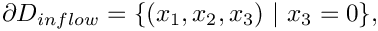and finally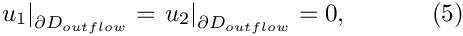(parallel flow) on the outflow boundary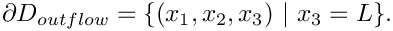Note that the axial velocity component,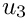, is not constrained at the outflow. Implicitly, we are therefore setting the axial component of traction on the fluid to zero,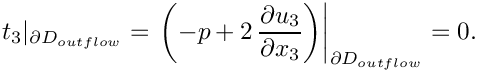Since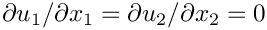in the outflow cross-section [see (5], and the flow is incompressible, this is equivalent to (weakly) setting the pressure at the outflow to zero,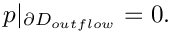## Results for Taylor-Hood elements

The figure below shows the results computed with oomph-lib's 3x3x3-node 3D adaptive Taylor-Hood elements for the parameters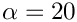,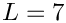and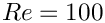. The large exponentimposes a very blunt inflow profile, which creates a thin boundary layer near the wall. Diffusion of vorticity into the centre of the tube smooths the velocity profile which ultimately approaches a parabolic Poiseuille profile. If you are viewing these results online you will be able to see how successive mesh adaptations refine the mesh – predominantly near the entry region where the large velocity gradients in the boundary layer require a fine spatial discretisation. Note also that on the coarsest mesh, even the (imposed) inflow profile is represented very poorly.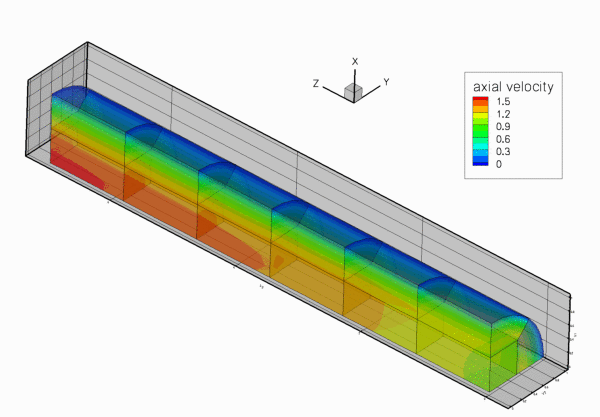Contour plot of the axial velocity distribution for Re=100. Flow is from right to left.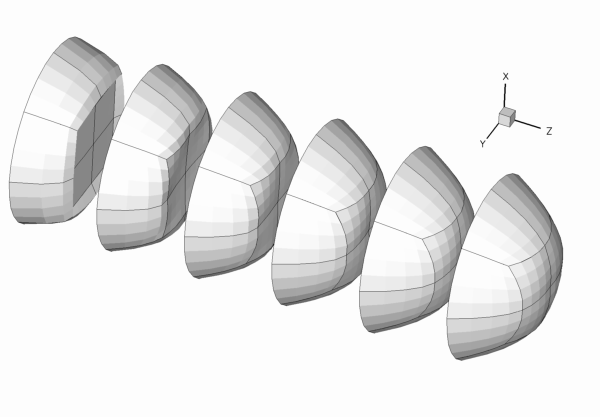Axial velocity profiles in equally-spaced cross-sections along the tube for Re=100. Flow is from left to right.

# Global parameters and functions

The problem only contains one global parameter, the Reynolds number, which we define in a namespace, as usual.

//=start_of_namespace================================================
/// Namespace for physical parameters
//===================================================================
{
/// Reynolds number
double Re=100;
} // end_of_namespace
Namespace for physical parameters.
Definition: full_tube.cc:83
double Re
Reynolds number.
Definition: full_tube.cc:85

# The driver code

Since the 3D computations can take a long time, and since all demo codes are executed during oomph-lib's self-test procedures, we allow the code to operate in two modes:

• By default, we specify error targets for which the code refines the mesh near the inflow region, and allow up to five successive mesh adaptations. The code is executed in this mode if the executable is run without any command line arguments.
• If the code is run during the self-test procedure (indicated by specifying some random command line argument), we only perform one level of adaptation to speed up the self-test. However, because the original mesh is very coarse, the first mesh adaptation refines all elements in the mesh (cf. the animation of the adaptive mesh refinement shown above), so that no hanging nodes are created – not a good test-case for a validation run! Therefore adjust the error targets so that the first (and only) mesh adaption only refines a few elements and therefore creates a few hanging nodes.

The main code therefore starts by storing the command line arguments and setting the adaptation targets accordingly:

//=start_of_main=======================================================
/// Driver for 3D entry flow into a quarter tube. If there are
/// any command line arguments, we regard this as a validation run
/// and perform only a single adaptation
//=====================================================================
int main(int argc, char* argv[])
{
// Store command line arguments
CommandLineArgs::setup(argc,argv);
// Allow (up to) five rounds of fully automatic adapation in response to
//-----------------------------------------------------------------------
// error estimate
//---------------
double max_error_target,min_error_target;
// Set max number of adaptations in black-box Newton solver and
if (CommandLineArgs::Argc==1)
{
// Error targets for adaptive refinement
max_error_target=0.005;
min_error_target=0.0005;
}
// Validation run: Only one adaptation. Relax error targets
// to ensure that not all elements are refined so we're getting
// some hanging nodes.
else
{
// Validation run: Just one round of adaptation
// Error targets for adaptive refinement
max_error_target=0.02;
min_error_target=0.002;
}
int main(int argc, char *argv[])
///////////////////////////////////////////////////////////////////// ///////////////////////////////...

We then create a DocInfo object to specify the labels for the output files, and solve the problem, first with Taylor-Hood and then with Crouzeix-Raviart elements, writing the results from the two discretisations to different directories:

// Set up doc info
DocInfo doc_info;
// Do Taylor-Hood elements
//------------------------
{
// Set output directory
doc_info.set_directory("RESLT_TH");
// Step number
doc_info.number()=0;
// Build problem
problem(doc_info,min_error_target,max_error_target);
cout << " Doing Taylor-Hood elements " << std::endl;
// Doc solution after solving
problem.doc_solution();
// Solve the problem
}
// Do Crouzeix-Raviart elements
//-----------------------------
{
// Set output directory
doc_info.set_directory("RESLT_CR");
// Step number
doc_info.number()=0;
// Build problem
problem(doc_info,min_error_target,max_error_target);
cout << " Doing Crouzeix-Raviart elements " << std::endl;
// Solve the problem
}
} // end_of_main
Entry flow problem in quarter tube domain.

# The problem class

The problem class is very similar to the ones used in the 2D examples. We pass the DocInfo object and the target errors to the Problem constructor.

//=start_of_problem_class=============================================
/// Entry flow problem in quarter tube domain
//====================================================================
template<class ELEMENT>
class EntryFlowProblem : public Problem
{
public:
/// Constructor: Pass DocInfo object and target errors
EntryFlowProblem(DocInfo& doc_info, const double& min_error_target,
const double& max_error_target);
/// Destructor (empty)
EntryFlowProblem(DocInfo &doc_info, const double &min_error_target, const double &max_error_target)
Constructor: Pass DocInfo object and target errors.
~EntryFlowProblem()
Destructor (empty)

The function Problem::actions_after_newton_solve() is used to document the solutions computed at various levels of mesh refinement:

/// Doc the solution after solve
void actions_after_newton_solve()
{
// Doc solution after solving
doc_solution();
// Increment label for output files
Doc_info.number()++;
}

The function Problem::actions_before_newton_solve() is discussed below, and, as in all adaptive Navier-Stokes computations, we use the function Problem::actions_after_adapt() to pin any redundant pressure degrees of freedom; see another tutorial for details.

/// Update the problem specs before solve
void actions_before_newton_solve();
/// After adaptation: Pin redudant pressure dofs.
{
// Pin redudant pressure dofs
RefineableNavierStokesEquations<3>::
pin_redundant_nodal_pressures(mesh_pt()->element_pt());
}

Finally, we have the usual doc_solution() function and include an access function to the mesh. The private member data Alpha determines the bluntness of the inflow profile.

/// Doc the solution
void doc_solution();
/// Overload generic access function by one that returns
/// a pointer to the specific mesh
RefineableQuarterTubeMesh<ELEMENT>* mesh_pt()
{
return dynamic_cast<RefineableQuarterTubeMesh<ELEMENT>*>(Problem::mesh_pt());
}
private:
/// Exponent for bluntness of velocity profile
int Alpha;
/// Doc info object
DocInfo Doc_info;
}; // end_of_problem_class

# The constructor

We start by building the adaptive mesh for the quarter tube domain. As for most meshes with curvilinear boundaries, the RefineablequarterTubeMesh expects the curved boundary to be represented by a GeomObject. We therefore create an EllipticalTube with unit half axes, i.e. a unit cylinder and a pass a pointer to the GeomObject to the mesh constructor. The "ends" of the curvilinear boundary (in terms of the maximum and minimum values of the Lagrangian coordinates that parametrise the shape of the GeomObject) are such that it represents a quarter of a cylindrical tube of length.

//=start_of_constructor===================================================
/// Constructor: Pass DocInfo object and error targets
//========================================================================
template<class ELEMENT>
const double& min_error_target,
const double& max_error_target)
: Doc_info(doc_info)
{
// Setup mesh:
//------------
// Create geometric objects: Elliptical tube with half axes = radius = 1.0
// Boundaries on object
Vector<double> xi_lo(2);
// height of inflow
xi_lo=0.0;
// start of Wall_pt
xi_lo=0.0;
Vector<double> xi_hi(2);
// height of outflow
xi_hi=7.0;
// end of Wall_pt
xi_hi=2.0*atan(1.0);
// # of layers
unsigned nlayer=6;
//Radial divider is located half-way along the circumference
double frac_mid=0.5;
// Build and assign mesh
Problem::mesh_pt()=
new RefineableQuarterTubeMesh<ELEMENT>(Wall_pt,xi_lo,frac_mid,xi_hi,nlayer);

Next, we build an error estimator and specify the target errors for the mesh adaptation:

// Set error estimator
Z2ErrorEstimator* error_estimator_pt=new Z2ErrorEstimator;
mesh_pt()->spatial_error_estimator_pt()=error_estimator_pt;
// Error targets for adaptive refinement
mesh_pt()->max_permitted_error()=max_error_target;
mesh_pt()->min_permitted_error()=min_error_target;

Now we have to apply boundary conditions on the various mesh boundaries. [Reminder: If the numbering of the mesh boundaries is not apparent from its documentation (as it should be!), you can use the function Mesh::output_boundaries(...) to output them in a tecplot-readable form.

//Doc the boundaries
ofstream some_file;
char filename;
sprintf(filename,"boundaries.dat");
some_file.open(filename);
mesh_pt()->output_boundaries(some_file);
some_file.close();

If the mesh has N boundaries, the output file will contain N different zones, each containing the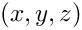coordinates of the nodes on the boundary.]

For the RefineableQuarterTubeMesh, the boundaries are numbered as follows:

• Boundary 0: "Inflow" cross section; located along the line parametrised by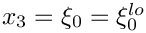on the GeomObject that specifies the wall.
• Boundary 1: Plane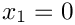• Boundary 2: Plane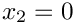• Boundary 3: The curved wall
• Boundary 4: "Outflow" cross section; located along the line parametrised byon the GeomObject that specifies the wall.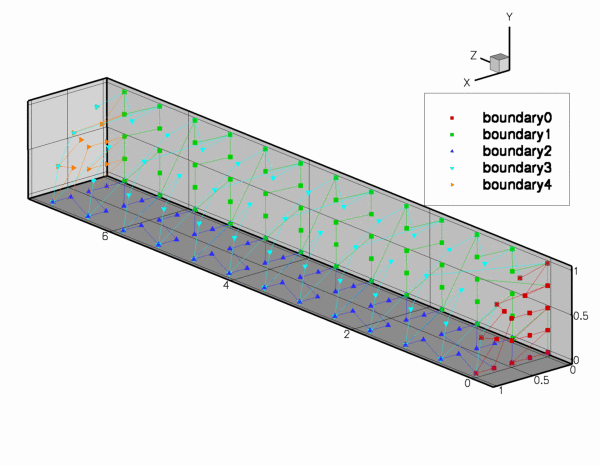Plot of the mesh boundaries.

We apply the following boundary conditions:

• Boundary 0: (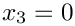) pin all three velocities.
• Boundary 1: () pin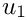.
• Boundary 2: () pin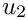.
• Boundary 3: (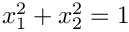) pin all three velocities.
• Boundary 4: (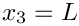) pinand.
// Set the boundary conditions for this problem: All nodal values are
// free by default -- just pin the ones that have Dirichlet conditions
// here.
unsigned num_bound = mesh_pt()->nboundary();
for(unsigned ibound=0;ibound<num_bound;ibound++)
{
unsigned num_nod= mesh_pt()->nboundary_node(ibound);
for (unsigned inod=0;inod<num_nod;inod++)
{
// Boundary 1 is the vertical symmetry boundary: We allow flow in
//the y-direction. Elsewhere, pin the y velocity
if(ibound!=1) mesh_pt()->boundary_node_pt(ibound,inod)->pin(1);
// Boundary 2 is the horizontal symmetry boundary: We allow flow in
//the x-direction. Elsewhere, pin the x velocity
if(ibound!=2) mesh_pt()->boundary_node_pt(ibound,inod)->pin(0);
// Boundaries 0 and 3 are the inflow and the wall respectively.
// Pin the axial velocity because of the prescribed inflow
// profile and the no slip on the stationary wall, respectively
if((ibound==0) || (ibound==3))
{
mesh_pt()->boundary_node_pt(ibound,inod)->pin(2);
}
}
} // end loop over boundaries

Now we assign the re_pt() for each element and pin the redundant nodal pressures (see another tutorial for details).

// Loop over the elements to set up element-specific
// things that cannot be handled by constructor
unsigned n_element = mesh_pt()->nelement();
for(unsigned i=0;i<n_element;i++)
{
// Upcast from GeneralisedElement to the present element
ELEMENT* el_pt = dynamic_cast<ELEMENT*>(mesh_pt()->element_pt(i));
//Set the Reynolds number, etc
el_pt->re_pt() = &Global_Physical_Variables::Re;
}
// Pin redudant pressure dofs
RefineableNavierStokesEquations<3>::
pin_redundant_nodal_pressures(mesh_pt()->element_pt());

We provide an initial guess for the velocity field by initialising all velocity components with their Poiseuille flow values.

// Set Poiseuille flow as initial for solution
// Inflow will be overwritten in actions_before_newton_solve()
unsigned n_nod=mesh_pt()->nnode();
for (unsigned j=0;j<n_nod;j++)
{
Node* node_pt=mesh_pt()->node_pt(j);
// Recover coordinates
double x=node_pt->x(0);
double y=node_pt->x(1);
double r=sqrt(x*x+y*y );
// Poiseuille flow
node_pt->set_value(0,0.0);
node_pt->set_value(1,0.0);
node_pt->set_value(2,(1.0-r*r));
}

Finally, we set the value of Alpha, the exponent that specifies the bluntness of the inflow profile and assign the equation numbers.

// Set the exponent for bluntness: Alpha=2 --> Poisseuille; anything
// larger makes the inflow blunter
Alpha=20;
//Attach the boundary conditions to the mesh
cout <<"Number of equations: " << assign_eqn_numbers() << std::endl;
} // end_of_constructor

# Actions before solve

We use the function Problem::actions_before_newton_solve() to re-assign the inflow boundary conditions before every solve. In the present problem this is an essential step because the blunt inflow profile (4) cannot be represented accurately on the initial coarse mesh (see the animation of the axial velocity profiles at the beginning of this document). As discussed in the example that illustrates the use of spatial adaptivity for time-dependent problems, oomph-lib automatically (i) applies the correct boundary conditions for newly created nodes that are located on the mesh boundaries and (ii) assigns the nodal values at such nodes by interpolation from the previously computed solution. This procedure is adequate on boundaries where homogeneous boundary conditions are applied, e.g. on the curved wall, the symmetry and the outflow boundaries. However, on the inflow boundary, the interpolation from the FE representation of the blunt velocity profile (imposed on the coarse initial mesh) onto the refined mesh, does not yield a more accurate representation of the prescribed inflow profile. It is therefore necessary to re-assign the nodal values on this boundary after every adaptation, i.e. before every solve.

//=start_of_actions_before_newton_solve==========================================
/// Set the inflow boundary conditions
//========================================================================
template<class ELEMENT>
{
// (Re-)assign velocity profile at inflow values
//--------------------------------------------
// Setup bluntish parallel inflow on boundary 0:
unsigned ibound=0;
unsigned num_nod= mesh_pt()->nboundary_node(ibound);
for (unsigned inod=0;inod<num_nod;inod++)
{
// Recover coordinates
double x=mesh_pt()->boundary_node_pt(ibound,inod)->x(0);
double y=mesh_pt()->boundary_node_pt(ibound,inod)->x(1);
double r=sqrt(x*x+y*y);
// Bluntish profile for axial velocity (component 2)
mesh_pt()->boundary_node_pt(ibound,inod)->
set_value(2,(1.0-pow(r,Alpha)));
}
void actions_before_newton_solve()
Update the problem specs before solve.

# Post processing

This function remains exactly the same as in the 2D examples.

//=start_of_doc_solution==================================================
/// Doc the solution
//========================================================================
template<class ELEMENT>
{
ofstream some_file;
char filename;
// Number of plot points
unsigned npts;
npts=5;
// Output solution
sprintf(filename,"%s/soln%i.dat",Doc_info.directory().c_str(),
Doc_info.number());
some_file.open(filename);
mesh_pt()->output(some_file,npts);
some_file.close();
} // end_of_doc_solution
void doc_solution()
Doc the solution.

1. Suppress the reassignment of the prescribed inflow profile in Problem::actions_before_newton_solve() to confirm that this step is essential if the computation is to converge to the exact solution.
2. Suppress the specification of the parabolic (Poiseuille) velocity profile as the initial guess for the velocity field in the problem constructor to confirm that the assignment of a "good" initial guess for the solution is essential for the convergence of the Newton method. [Hint: You can simply comment out the initialisation of the velocities – they then retain their default initial values of 0.0. When you re-run the code, the Newton iteration will "die" immediately with an error message stating that the maximum residual exceeds the default threshold of 10.0, stored in the protected data member Problem::Max_residuals. Try increasing the value of this threshold in the Problem constructor. Is this sufficient to make the Newton method converge?]